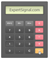Average Directional Movement Index

Usage: Analyzing Market Conditions

Standard Settings: 14 PeriodsIntroduction to the Directional Movement System

The Directional Movement System was introduced by Welles Wilder in his book “New Concepts in Technical Trading Systems”. What distinguishes Directional Movement System from other systems is that it first evaluates if a market is trending before offering trading signals. The default settings for the indicator involves 14 periods.

The Directional Movement System consists three lines:

(a) The Positive Direction Indicator (+DI) which summarizes the upward trend movement

(b) The Negative Direction Indicator (-DI) which summarizes the downward trend movement

(c) The Average Directional Movement Index (ADX) which indicates whether the market is trending or rangingCalculating the Directional Movement System

■ First we must calculate the today’s Directional movement

+DM = Today's High - Yesterday's High (when the market moves upward)
-DM = Yesterday's Low - Today's Low (when the market moves downward)

■ After we calculate the True Range for the day, as follows:

Today's High - Today's Low
Today's High - Yesterday's Close
Yesterday's Close - Today's Low

■ Next, we calculate +DM14, as follows:

+DM14 = exponential moving average of +DM

■ Next, we calculate -DM14

-DM14 = exponential moving average of -DM

■ Next, we calculate TR14

TR14 = exponential moving average of True Range

■ After we calculate the Directional Indicators:

+DI14 = +DM14 / TR14

-DI14 = -DM14 / TR14

■ Finally, we calculate the parts of ADX (Average Directional Movement Index)

Calculate the DI Difference (+DI14 and -DI14) as a positive number.

■ Calculate the Directional Index (DX):

DX = DI Difference / (sum of +DI14 and -DI14)

ADX = the exponential moving average of DXTrading with the Average Directional Movement Index (ADX)

□ +DI summarizes the upward trend movement and -DI summarizes the downward trend movement

□ If ADX declines that is a signal that the market is losing direction

□ If the value of ADX moves below both +DI and -DI then that is a very bad signal regarding the upcoming market conditions

□ The more time ADX remains below both +DI and -DI then the stronger the new trend will emerge

□ If ADX moves above both +DI and -DI then it is a signal that the market becomes overbought

□ It is better to wait until ADX rises 4 steps off its low and then trade (for example if the low=17 wait until ADX=21)Platform Setup

□ GO TO → INDICATORS → TREND → AVERAGE DIRECTIONAL MOVEMENT INDEX

□ SETTINGS → 14 PERIODS